# Helioprojective#

class sunpy.coordinates.frames.Helioprojective(*args, **kwargs)[source]#

A coordinate or frame in the Helioprojective Cartesian (HPC) system, which is observer-based.

• The origin is the location of the observer.

• Tx (aka “theta_x”) is the angle relative to the plane containing the Sun-observer line and the Sun’s rotation axis, with positive values in the direction of the Sun’s west limb.

• Ty (aka “theta_y”) is the angle relative to the Sun’s equatorial plane, with positive values in the direction of the Sun’s north pole.

• distance is the Sun-observer distance.

This system is frequently used in a projective form without distance specified. For observations looking very close to the center of the Sun, where the small-angle approximation is appropriate, Tx and Ty can be approximated as Cartesian components.

A new instance can be created using the following signatures (note that if supplied, obstime and observer must be keyword arguments):

Helioprojective(Tx, Ty, obstime=obstime, observer=observer)
Helioprojective(Tx, Ty, distance, obstime=obstime, observer=observer)

Parameters:

Examples

>>> from astropy.coordinates import SkyCoord
>>> import sunpy.coordinates
>>> import astropy.units as u
>>> sc = SkyCoord(0*u.deg, 0*u.deg, 5*u.km,
...               obstime="2010/01/01T00:00:00", observer="earth", frame="helioprojective")
>>> sc
<SkyCoord (Helioprojective: obstime=2010-01-01T00:00:00.000, rsun=695700.0 km, observer=<HeliographicStonyhurst Coordinate for 'earth'>): (Tx, Ty, distance) in (arcsec, arcsec, km)
(0., 0., 5.)>
>>> sc = SkyCoord(0*u.deg, 0*u.deg,
...               obstime="2010/01/01T00:00:00", observer="earth", frame="helioprojective")
>>> sc
<SkyCoord (Helioprojective: obstime=2010-01-01T00:00:00.000, rsun=695700.0 km, observer=<HeliographicStonyhurst Coordinate for 'earth'>): (Tx, Ty) in arcsec
(0., 0.)>
>>> sc = SkyCoord(CartesianRepresentation(1*u.AU, 1e5*u.km, -2e5*u.km),
...               obstime="2011/01/05T00:00:50", observer="earth", frame="helioprojective")
>>> sc
<SkyCoord (Helioprojective: obstime=2011-01-05T00:00:50.000, rsun=695700.0 km, observer=<HeliographicStonyhurst Coordinate for 'earth'>): (Tx, Ty, distance) in (arcsec, arcsec, AU)
(137.87948623, -275.75878762, 1.00000112)>


Attributes Summary

 angular_radius Angular radius of the Sun as seen by the observer. default_differential Default representation for differential data (e.g., velocity) default_representation Default representation for position data frame_attributes frame_specific_representation_info Mapping for frame-specific component names name observer rsun

Methods Summary

 assume_spherical_screen(center[, only_off_disk]) Context manager to interpret 2D coordinates as being on the inside of a spherical screen. This method calculates the third coordinate of the Helioprojective frame.

Attributes Documentation

Angular radius of the Sun as seen by the observer.

The rsun frame attribute is the radius of the Sun in length units. The tangent vector from the observer to the edge of the Sun forms a right-angle triangle with the radius of the Sun as the far side and the Sun-observer distance as the hypotenuse. Thus, the sine of the angular radius of the Sun is ratio of these two distances.

default_differential#

Default representation for differential data (e.g., velocity)

default_representation#

Default representation for position data

frame_attributes = {'observer': <sunpy.coordinates.frameattributes.ObserverCoordinateAttribute object>, 'obstime': <sunpy.coordinates.frameattributes.TimeFrameAttributeSunPy object>, 'rsun': <astropy.coordinates.attributes.QuantityAttribute object>}#
frame_specific_representation_info#

Mapping for frame-specific component names

name = 'helioprojective'#
observer = None#
rsun = <Quantity 695700. km>#

Methods Documentation

classmethod assume_spherical_screen(center, only_off_disk=False)[source]#

Context manager to interpret 2D coordinates as being on the inside of a spherical screen.

The radius of the screen is the distance between the specified center and Sun center. This center does not have to be the same as the observer location for the coordinate frame. If they are the same, then this context manager is equivalent to assuming that the helioprojective “zeta” component is zero.

This replaces the default assumption where 2D coordinates are mapped onto the surface of the Sun.

Parameters:

Examples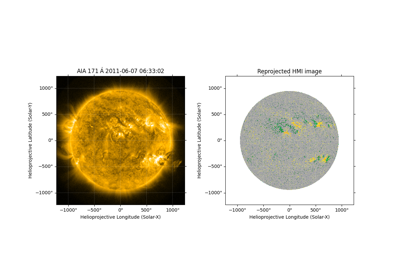Aligning AIA and HMI Data with Reproject

Aligning AIA and HMI Data with Reproject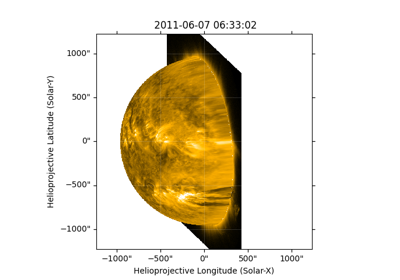Reprojecting Using a Spherical Screen

Reprojecting Using a Spherical ScreenBlending maps using mplcairo

Blending maps using mplcairo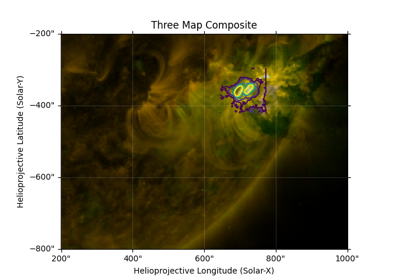Creating a Composite Plot with Three Maps

Creating a Composite Plot with Three Maps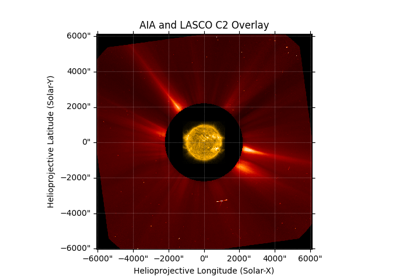Overlay an AIA image on a LASCO C2 coronagraph

Overlay an AIA image on a LASCO C2 coronagraph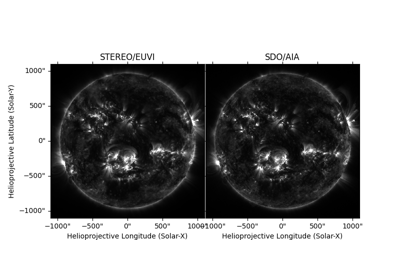Visualizing 3D stereoscopic images

Visualizing 3D stereoscopic images
>>> import astropy.units as u
>>> from sunpy.coordinates import Helioprojective
>>> h = Helioprojective(range(7)*u.arcsec*319, *7*u.arcsec,
...                     observer='earth', obstime='2020-04-08')
>>> print(h.make_3d())
<Helioprojective Coordinate (obstime=2020-04-08T00:00:00.000, rsun=695700.0 km, observer=<HeliographicStonyhurst Coordinate for 'earth'>): (Tx, Ty, distance) in (arcsec, arcsec, AU)
[(   0., 0., 0.99660825), ( 319., 0., 0.99687244),
( 638., 0., 0.99778472), ( 957., 0., 1.00103285),
(1276., 0.,        nan), (1595., 0.,        nan),
(1914., 0.,        nan)]>

>>> with Helioprojective.assume_spherical_screen(h.observer):
...     print(h.make_3d())
<Helioprojective Coordinate (obstime=2020-04-08T00:00:00.000, rsun=695700.0 km, observer=<HeliographicStonyhurst Coordinate for 'earth'>): (Tx, Ty, distance) in (arcsec, arcsec, AU)
[(   0., 0., 1.00125872), ( 319., 0., 1.00125872),
( 638., 0., 1.00125872), ( 957., 0., 1.00125872),
(1276., 0., 1.00125872), (1595., 0., 1.00125872),
(1914., 0., 1.00125872)]>

>>> with Helioprojective.assume_spherical_screen(h.observer, only_off_disk=True):
...     print(h.make_3d())
<Helioprojective Coordinate (obstime=2020-04-08T00:00:00.000, rsun=695700.0 km, observer=<HeliographicStonyhurst Coordinate for 'earth'>): (Tx, Ty, distance) in (arcsec, arcsec, AU)
[(   0., 0., 0.99660825), ( 319., 0., 0.99687244),
( 638., 0., 0.99778472), ( 957., 0., 1.00103285),
(1276., 0., 1.00125872), (1595., 0., 1.00125872),
(1914., 0., 1.00125872)]>

make_3d()[source]#

This method calculates the third coordinate of the Helioprojective frame. It assumes that the coordinate point is on the surface of the Sun.

If a point in the frame is off limb then NaN will be returned.

Returns:

new_frame (Helioprojective) – A new frame instance with all the attributes of the original but now with a third coordinate.